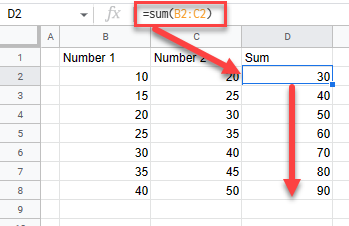# Apply Formula to Entire Column (Copy Down) – Excel & Google Sheets

This tutorial demonstrates how to apply a formula to an entire column in Excel and Google Sheets.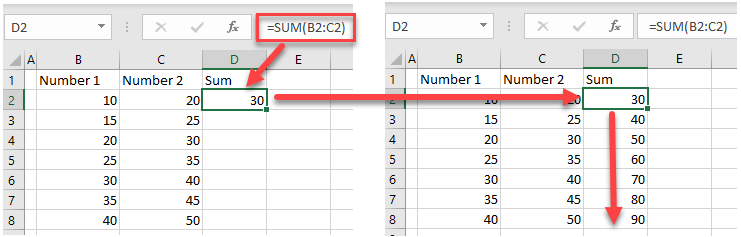## Fill Formula Down Entire Column

### Drag Fill Handle

Say you have a formula in cell D2 that sums values from Columns B and C.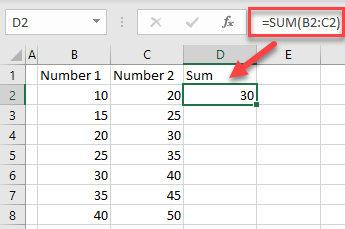1. To apply the same formula to the entire column (through Row 8), position the cursor in the bottom-right corner of the cell containing the formula (D2).
2. Then, drag it to the end of the column (Row 8).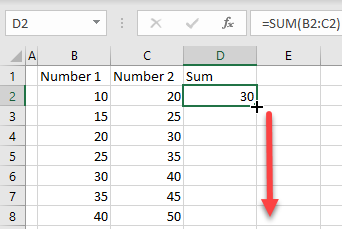As a result, the SUM formula is copied to the rest of cells in the Column D.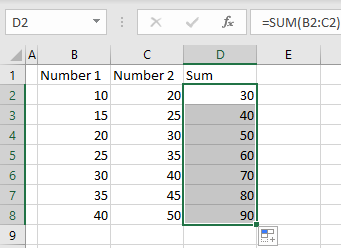### Double-Click Fill Handle

Alternatively, you can double-click the fill handle to apply the formula to the entire column.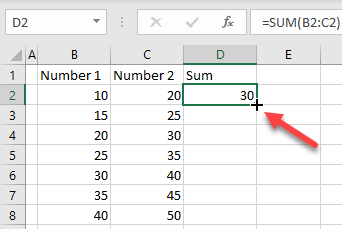In this case, the last row for copying the formula is determined by the number of rows in the adjacent column. Since Column C has eight rows populated, the formula in Column D is applied through Row 8.## Copy-Paste Formula

Another approach for copying the formula is to use the copy and paste functionality.

1. Right-click the cell from which you want to copy the formula (D2), and choose Copy (or use the keyboard shortcut CTRL + C).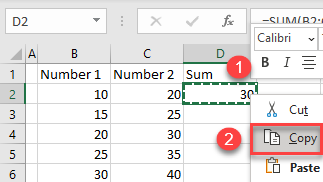1. On your keyboard, press CTRL + SHIFT + END to select the paste range, right-click the selected area, and choose Paste (or use the keyboard shortcut CTRL + V).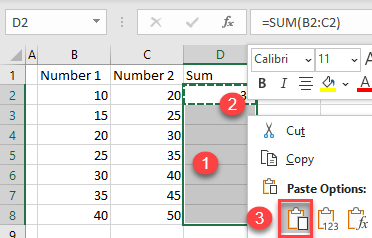As a result, the formula is copied to the rest of Column D.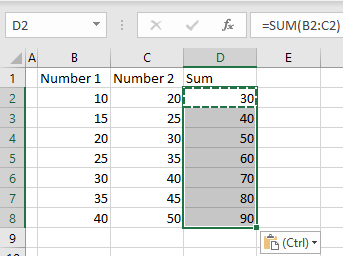#### Tips:

• You can use CTRL + SHIFT + END in this example because the column with the formula is the last one populated. However, if there were more columns on the right side, Excel wouldn’t have selected properly, and you would need to select the range manually. The selection here is made based on the number of rows in the left adjacent column (through Row 8).
• There is also the CTRL + ↓ (down arrow) shortcut, which selects the entire column under the selected cell down to the first populated row. In this case, since you don’t have any cells populated under D2, this shortcut selects all available rows in Column D (1,048,576).
• If the formula you want to copy down is not at the beginning of the column, but somewhere in the middle, you can use CTRL + SPACE to select an entire column and paste the formula.

## Apply Formula to Entire Column in Google Sheets

Except for CTRL + SHIFT + END, all other methods to apply a formula to an entire column can be used in Google Sheets as well.